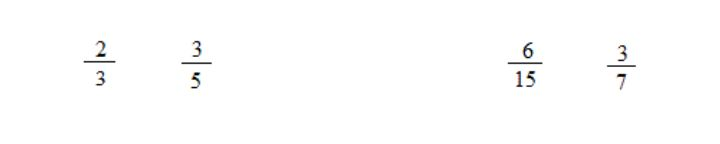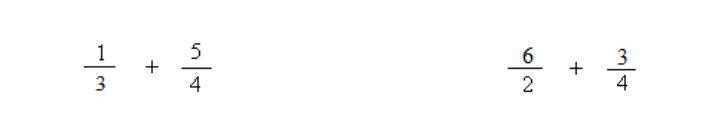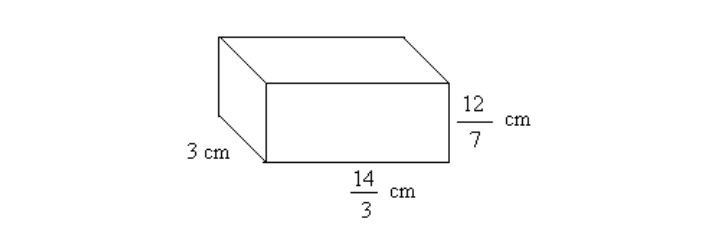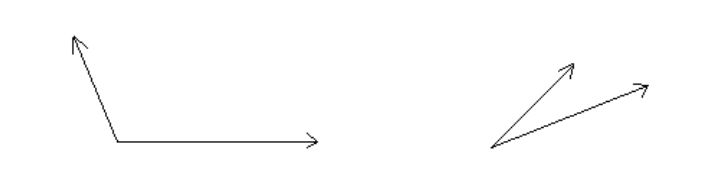# Basic Math Diagnostic Test

Math TestsA basic math diagnostic test can assess and evaluate a student’s strengths and areas for improvement in mathematics using a simple math exam. Students in elementary school may improve their scores by studying the material that will be on the mathematics assessment test and then taking practice tests. If you’re a student studying for a math test or an adult wanting to brush up on your math abilities, this article will provide the information and tools you need to do well.

## The Importance of Diagnostic Tests

The math diagnostic test is necessary for teachers to evaluate student’s math abilities and grasp key math concepts. In this way, instructors may better tailor their lessons to the individual requirements of their students by focusing on the areas in which they know their students are struggling.

Students who do very well on a math evaluation test might be flagged for possible promotion to more difficult courses. To help these kids go more quickly through key mathematical topics, instructors might target their instruction more specifically.

You can get a math diagnostic test free and also use it to measure learning and evaluate teachers’ performance. Teachers may gauge whether or not their approaches are having the intended effect on their students’ learning by giving the same exam at many junctures. It’s useful for pinpointing places that might want some tweaking or improvement.

Math assessments help teachers, students, and parents identify and address students’ specific math strengths and weaknesses. By recognizing and working with these issues in their formative years, kids can strengthen their mathematical competence and self-assurance.

However, a diagnostic math assessment test shouldn’t be used as the only gauge of a student’s mathematical competence or development. To gain a more accurate picture of a student’s strengths and areas for progress, they should be used in conjunction with other types of assessment, such as classwork, homework, and projects.

## Math Diagnostic Test

The purpose of the math assessment online is to get a sense of how comfortable a person is with the most fundamental aspects of mathematics. Elementary math assessment is typically taken to elementary and middle school kids to measure their mathematical skills and identify any areas of weakness that need to be addressed. The math assessment free idea is also administered to adults who want to brush up on their arithmetic knowledge or need to prove their competency in the subject for professional purposes.

Timed tests in free diagnostic math assessment elementary like arithmetic, algebra, and geometry can be given to students. The four fundamental mathematical operations—addition, subtraction, multiplication, and division—are the subject of arithmetic tests. The ability to solve equations and work with variables is what algebra tests are looking for.

Multiple-choice, fill-in-the-blank, and short-answer questions are all part of the math assessment. Depending on the examiner’s preference, the high school math diagnostic test may be timed or untimed.

You may print off this little online math assessment free review before starting.

1. Convert the number below to words:

157802  __________

2. Round 36,993 to the nearest thousands __________
3. Round 6,724 to the nearest hundredth __________
4. 324 multiplied by 87 is __________
5. 81.32 multiplied by 0.3214 is __________
6. Divide 4356 by 89; the answer is __________
7. What is the highest common factor (HCF) of 24 and 76 __________
8. What is the least common factor (LCF) of 12 and 36 __________
9. Detect the prime numbers below and circle them.

2       5       8       13       17       18       21       93       117

10. Determine if the fraction on the left is less or greater than the fraction on the right. Use < or > to indicate your answer11. Sum up the following fractions:12. Determine if the decimal on the left is less or greater than the decimal on the right. Use < or >

5.43123        5.43013

13. Find the perimeter and area of a rectangle with sides equal to 5 inches and 7 inches?

Perimeter = __________ inches       area=__________

14. 35% of 40 is __________
15. The percent of 80 in 100 is what? __________
16. As a percent, ⅖ is __________
17. In your own words, describe what it means for two lines to be parallel. Make a drawing.
18. Find n: 6n + 12 = 6
19. Find the volume of the following figure? __________20. Find how much time has passed between 5:45 AM and 4:10 PM.
21. Using a protractor, measure the following angles __________22. Find the perimeter and area of a circle with a diameter equal to 5 cm?

Perimeter =__________     area=__________

23. 25 cm =__________mm        1250 g =__________ kg        25 mL = __________L
24. Convert the difference between 25 min. 14 sec and 4 min. 30 sec into seconds__________
25. The drawing of a car is made on a scale of 1:20. What is the car’s real length if the drawing is 10 inches?

Real length__________

26. In scientific notation, write these numbers

2300000000__________             0.00024__________

27. 12 feet = __________ yards            2 gallons = __________ pints
28. -2 + -6 =________            -4 + 3 = _________            5 − – 2 = _________
29. -2 × 4 =__________          -6 ÷ – 2=__________
30. Find the absolute value of -3?

## Printable Math Assessment Test for Different Grades

Many printable math assessment tests and online math assessment solutions are available for various grade levels. Teachers, parents, and students alike may evaluate the kids’ arithmetic understanding and proficiency with the use of online grade calculators. It’s important to remember that these tests are meant for elementary math diagnostic test purposes only and not as a determinant of a student’s mathematical prowess.

## What Math Skills Are Tested in the Basic Math Test?

A math diagnostic assessment free focus on particular mathematical abilities may shift based on the test’s context and target population. A free basic math skills assessment printable typically assesses knowledge in the following areas of mathematics:

### Basic arithmetic skills

Addition, subtraction, multiplication, and division are all part of basic arithmetic, as are an understanding of place value and the proper sequence of operations.

### Fractions

Mathematical operations using fractions include simplifying, adding, subtracting, and converting them to and from decimals.

### Decimals

Operations with decimals include arithmetic with decimals, division by decimals, multiplication by decimals, and division by fractions.

### Percentages

Basic math assessment problems involving percentages may be solved by using techniques like finding a percentage of a number, determining the percentage rise or decrease between two numbers, and so on.

### Basic Geometry

Some examples of what may be considered “basic geometry” include being able to recognize and sort various objects into categories, learning and using simple geometric terms (such as lines, angles, and sides), and solving simple geometric equations.

### Fundamental Algebra

Variables, equations, and the graphing of linear equations are all potential topics for a first course in algebra.

## How to Prepare for a Math Test?

If you have a math test coming up, here are some free online math diagnostic test tips to help you ace it:

### Review the Material

You should study the math topics that will be on the free math screening assessments until you are certain that you have a firm grasp of them. Read over your class materials (notes, textbook, etc.) again.

### Practice Problems

Get a feel for the test’s format by working through a free math diagnostic test. You should prepare for the test by becoming used to the structure and style of the questions.

### Time Yourself

Learn how long each kind of diagnostic math assessment elementary problem takes you to solve by timing yourself as you go through examples. It will help you pace yourself throughout the actual exam.

### Review Key Formulas and Concepts

Verify that you have mastered the ideas and formulae on the exam.

### Take Breaks

Get plenty of rest the night before the exam by spacing out your studying and taking breaks. It’s a great way to keep your mind fresh and prevent burnout.

### Seek Help

If you need math help with the content of free math assessments, contact your instructor or a tutor for guidance. They may give further assistance and explain any issues you may need help with.

Following the strategies in the free diagnostic math assessment may put you up for success on your math exam.

## Look Online for More Math Help

If your kid is experiencing problems with this diagnostic test, request Brighterly‘s evaluation lesson. With Brighterly, your kid has access to a wealth of math diagnostic test online materials, such as video lessons, quizzes, and games, to help them ace their next math test. Since information on free math assessment online is accessible 24/7 from any device with an internet connection, this provides your kid with great freedom and convenience.

Students who might benefit from diagnostic math assessment help can save money by using Brighterly’s online math tutoring rather than paying for private lessons with a tutor in person. Brighterly’s individualized lesson plans help your kid strengthen the skills in which they are weakest. It works better than the standard classroom model, in which pupils are expected to study the same basic math assessment test regardless of their particular requirements.

After-School Math Programs• Our program for 1st to 8th grade students is aligned with School Math Curriculum.

After-School Math Programs
Our program for 1st to 8th grade students is aligned with School Math Curriculum.